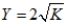# [Solved] An Economy Has a Capital Stock of 100

Question 130
Essay

## An economy has a capital stock of 100. The economy's labor, technology, and education levels are constant, and the economy has a production function that can be described by .The depreciation rate can be described by the linear function depreciation = 0.02K. Suppose the initial investment rate is investment = 0.2 and then some foreign savings start flowing into the economy. This investment function then changes to 0.3. By how much does the steady state capital stock change? By how much does output change? Show all your calculations.

10+ million students use Quizplus to study and prepare for their homework, quizzes and exams through 20m+ questions in 300k quizzes.

Explore our library and get Economics Homework Help with various study sets and a huge amount of quizzes and questions

3.5K

Study sets

66.3K

Quizzes

5.9M

Questions

Upload material to get free accessInvite a friend and get free accessSubscribe and get an instant access